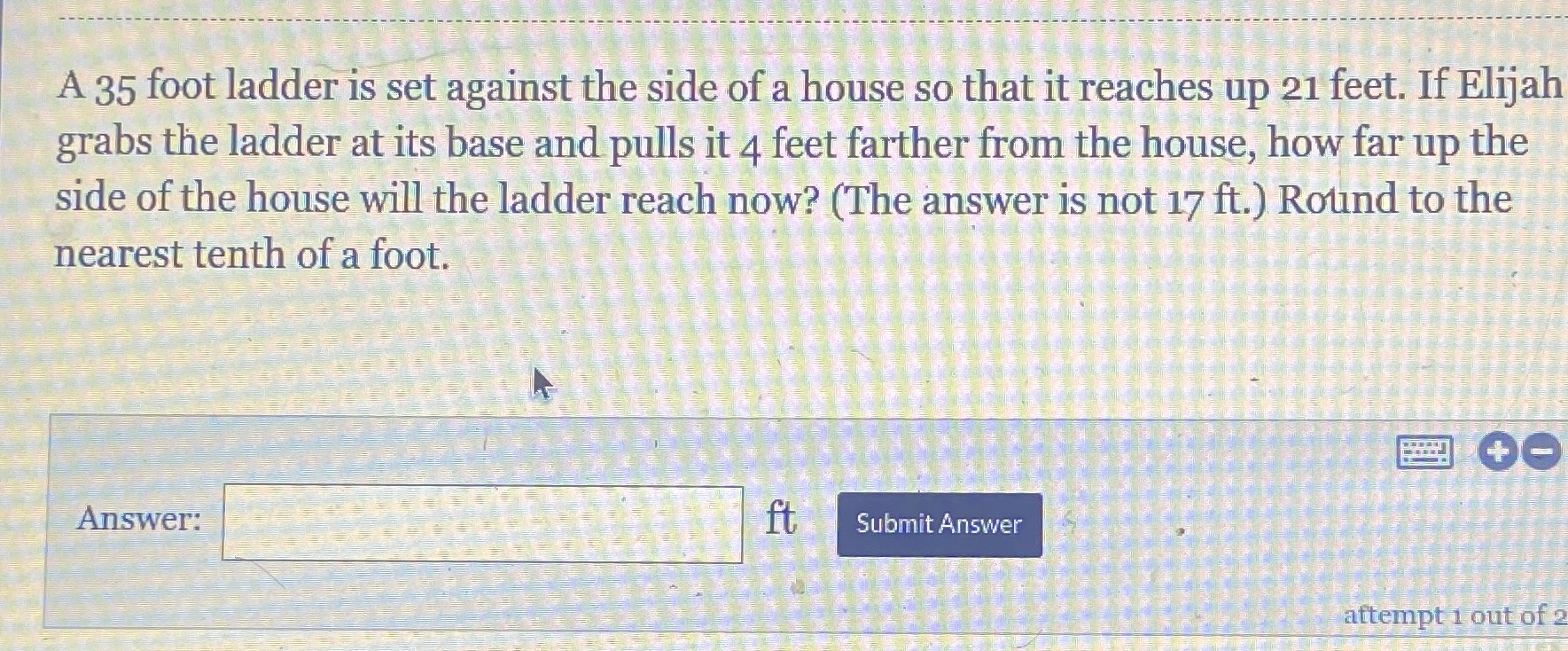### ¿Todavía tienes preguntas de matemáticas?

Pregunte a nuestros tutores expertos
Algebra
Pregunta$$35$$ foot ladder is set against the side of a house so that it reaches up $$21$$ feet. If Elijah grabs the ladder at its base and pulls it $$4$$ feet farther from the house, how far up the side of the house will the ladder reach now? (The answer is not $$17 ft$$ .) Round to the nearest tenth of a foot.

$$14.2$$ $$foot$$